# NCERT Solutions for Class 12 Geography Chapter 2 - Data Processing

##### Question 1:

The measure of central tendency that does not get affected by extreme values.

1. Mean
2. Mean and Mode
3. Mode
4. Median

(d) Median

##### Question 2:

The measure of central tendency always coinciding with the hump of any distribution is

1. Median
2. Median and Mode
3. Mean
4. Mode

(b) Median and Mode

##### Question 3:

A scatter plot represents negative correlation if the plotted values run from

1. Upper left to lower right
2. Lower left to upper right
3. Left to right
4. Upper right to lower left

(a) Upper left to lower right

##### Question 4:

Define the mean.

Mean or average is a quotient of the sum of several quantities. It is a number that by and large represents a series of numbers so, average can be taken as the central value or tendency of the population, in this case, all the students of your class.

##### Question 5:

What are the advantages of using mode?

Advantages of using mode are –

1. It is easy to understand and simple to calculate.
2. It is not affected by extremely large or small values.
3. It can be located first by inspection in ungrouped data and discrete frequency distribution.
4. It can be useful for qualitative data.
5. It can be computed in an open end frequency table.
##### Question 6:

What is dispersion?

Dispersion refers to the scattering of scores about measure of central tendency. Dispersion is also used to measure the extent to which individual items or numerical data tend to vary or spread about an average value. So, dispersion is the degree of spread or scatter or variation of measures about a central value.

##### Question 7:

Define correlation.

Two variables are said to be correlated if the change of one variable results in a corresponding change in the other variable. The first variable causing a change of second variable is known as independent variable (X) and the other variable is known as dependent variable (Y). As estimation of the strength and direction of the association between two variables is called simple or bivariate correlation.

##### Question 8:

What is perfect correlation?

Perfect correlation is a relationship between two variables in which both variables move in tandem. A positive correlation exists when one variable decreases as the other variable decreases, or one variable increases while the other increases. The maximum degree of correspondence or relationship goes up to 1 tone in mathematical terms. On adding an element of the direction of correlation it spreads the maximum extent of –1 to +1 through zero. It can never be more than one correlation if 1 is known as perfect correlation.

##### Question 9:

What is the maximum extent of correlation?

The maximum extent of correlation is 1(one) in mathematical term. It can never be more than one.

##### Question 10:

Explain relative position of mean, median and mode in a normal distribution and skewed distribution with the help of diagrams.

The three measures mean, median and mode of the central tendency could easily be compared with the help of normal distribution curve which is given below.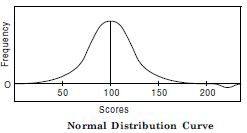Normal Distribution Curve
The normal distribution has an important characteristic. The mean, median, mode are the same score because a normal distribution is symmetrical. The score with the highest frequency occurs in the middle of the distribution and exactly half of the scores occur above the middle and half of the scores occur below. Most of the scores occur around the middle of the distribution of the mean. Very high and very low scores do not occur frequently and are, therefore, considered rare.
If the data are skewed or distorted in some way the mean, median and mode will not coincide and the effect of the skewed data needs to be considered in the following figure.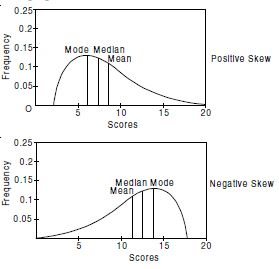##### Question 11:

Comment on the applicability of mean, median and mode. (Hint from their merits and demerits)

Mean, median and mode are applicable as their advantages are–

1. Simplicity: This is simple of all the measures of central tendency.
2. Representative value: This is based on the items in a series and is, therefore, a representative value of different items.
3. Certainty: Arithmetic mean is a value. It has no scope for estimated values.
4. Stability: Arithmatic mean has a stable value. The changes in he values of samples do not effect it.
5. Fair values: It balances the values of entire distribution.

Median:

1. This is difinite.
2. Median is easy to calculate and understand median.
3. Median can also be determined graphically.
4. It is not affected in the absence of anyone of the item.
5. It is helpful in qualitative facts such as ability, stability etc.

Mode:

1. Mode is simple precise and easy to understand.
2. It can be located on graph.
3. It cannot be given further mathematical treatment.
4. This is not affected by extreme items becasue it is not based on every item of the series.
##### Question 12:

Explain the process of computing standard deviation with the help of an in aginary example.

Standard deviation is the square root of the arithmetic mean of the square of deviation of the items from their mean value. It is precise measure of dispersion and is denoted by a greek letter (small sigma)

Computation of Standard Deviation–
Following formula is used to calculate the standard deviation for ungrouped data: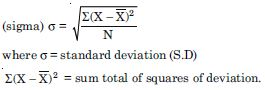N = Number of items.
The above formula becomes rather tendious if the value of X involves decimal points and also if the number of observations is very large. We may then use following short cut method: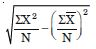Example – Following table shows the rainfall figures of last ten years. Calculate the standard deviation.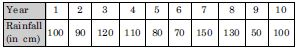##### Question 13:

Which measure of dispersion is the most unstable statistic and why?

It can be observed that the mean derived from the two data sets given as under is same i.e. 50. The highest and the lowest score shown as above table.
A 55 and 45 respectively. The distribution in table B has a high score of 98 and a low score of zero. The range of first distribution is 10 whereas it is 98 in the second distribution. Although the mean for both the groups is same, the first group is obviously stable or homogenous as compared to the distribution of score of the second group which is highly unstable or heterogeneous.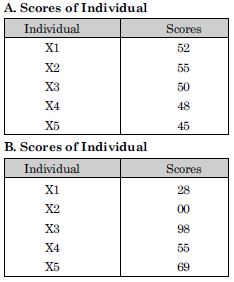##### Question 14:

Write a detailed note on the degree of correlation.

When reference has been made about the direction of correlation, negative or positive a natural curiosity arises to know the degree of correspondence or association of the two variables. The maximum degree of correspondence or relationship goes upto 1 (one) in mathematical terms. It can never be more than one. Correlation of 1 is known as perfect correlation (whether positive and negative). Between the two points of divergent perfect correlation lies 0 (zero) correlation a point of correlation or absence of any correlation between the variables.

##### Question 15:

What are various steps for the calculation of rank order correlation?

The following steps are as under for the calualtion of rank order correlation:

1. Copy the data related to X–Y variable given in the excercise and put them in the first and second column of the table.
2. Both the variables are to be ranked separately. The ranks of X-variable are to be recorded in third column headed by XR (Rank-x). Similarly the ranks of Y-variables (YR) are to be recorded in the fourth column.
3. Now since both XR and YR have been obtained find the difference between two sets of ranks and record it in the fifth column.
4. Each of these difference is squared and sum of the column of square is obtained. This value is placed in sixth column.
5. Then the computation of the rank correlation is done by the application of the following equation: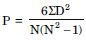Where P = Rank correlation
SD2 = Sura of the squares of the difference between two sets of ranks.
N = The number of Pairs of X–Y.